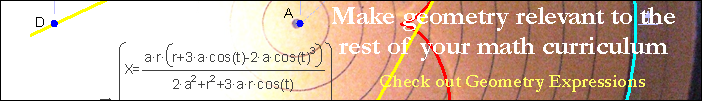Segment Area in Degrees

Download Math Illustrations File

The area of a segment is equal to the area of the sector reduced by the area of the triangle formed by the chord and two radii. It is convenient to use the triangle area formula A = 1/2 ab sin C, which becomes the form seen here.Drag A, B, and C to modify the diagram.

 Download As: